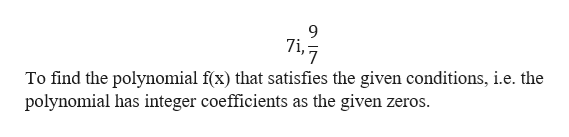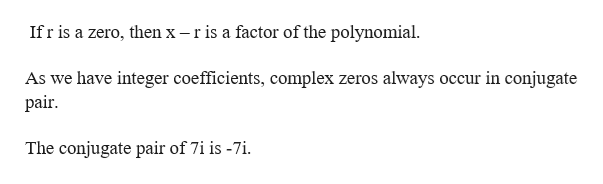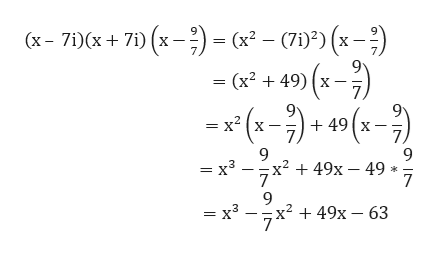# White a polynomial f(x) that satisfies the given conditions.Degree 3 polynomial with integer coefficients with zeros 7i and 9/7.

Question
39 views

White a polynomial f(x) that satisfies the given conditions.

Degree 3 polynomial with integer coefficients with zeros 7i and 9/7.

check_circle

Step 1

Given zeros of a polynomial,help_outlineImage Transcriptionclose7i, To find the polynomial f(x) that satisfies the given conditions, i.e. the polynomial has integer coefficients as the given zeros fullscreen
Step 2

Note:help_outlineImage TranscriptioncloseIfr is a zero, then x -ris a factor of the polynomial As we have integer coefficients, complex zeros always occur in conjugate pair The conjugate pair of 7i is -7i fullscreen
Step 3

Thus, we multiply all...help_outlineImage Transcriptionclose(x- 7i)(x+71) (x = (x- C7i)) (x) - (x + 49) (x7 (x-749 (x7) x2 = = x3 -x2 + 49x - 49 7 = x3 x2 49x - 63 fullscreen

### Want to see the full answer?

See Solution

#### Want to see this answer and more?

Solutions are written by subject experts who are available 24/7. Questions are typically answered within 1 hour.*

See Solution
*Response times may vary by subject and question.
Tagged in

### Equations and In-equations